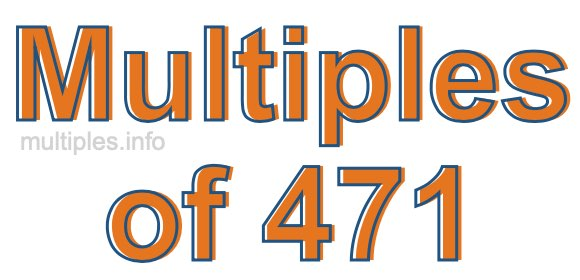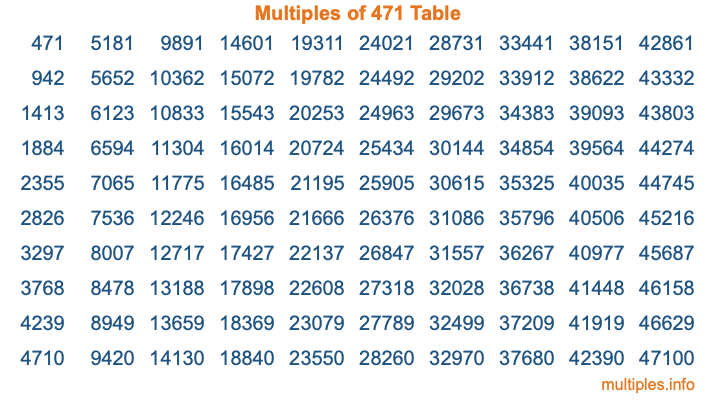Multiples of 471Welcome to the Multiples of 471 page. Here we will first teach you everything you will ever need to know about the multiples of 471, and then give you a study guide summary of everything we taught you to make sure you remember it all. Use this page to look up facts and learn information about the multiples of 471. This page will make you a multiples of four hundred seventy-one expert!

Definition of Multiples of 471
Multiples of 471 are all the numbers that when divided by 471 equal an integer. Each of the multiples of 471 are called a multiple. A multiple of 471 is created by multiplying 471 by an integer.

Therefore, to create a list of multiples of 471, you start with 1 multiplied by 471, then 2 multiplied by 471, then 3 multiplied by 471, and so on for as long as you want. Thus, the list of the first five multiples of 471 is 471, 942, 1413, 1884, and 2355. To see a larger list of multiples of 471, see the printable image of Multiples of 471 further down on this page. We also have a category where you can choose any nth multiple of 471.

Multiples of 471 Checker
The Multiples of 471 Checker below checks to see if any number of your choice is a multiple of 471. In other words, it checks to see if there is any number (integer) that when multiplied by 471 will equal your number. To do that, we divide your number by 471. If the the quotient is an integer, then your number is a multiple of 471.

Is  a multiple of 471?

Least Common Multiple of 471 and ...
A Least Common Multiple (LCM) is the lowest multiple that two or more numbers have in common. This is also called the smallest common multiple or lowest common multiple and is useful to know when you are adding our subtracting fractions. Enter one or more numbers below (471 is already entered) to find the LCM.

Check out our LCM Calculator if you need more details about the Least Common Multiple or if you need the LCM for different numbers for adding and subtraction fractions.

nth Multiple of 471
As we stated above, 471 is the first multiple of 471, 942 is the second multiple of 471, 1413 is the third multiple of 471, and so on. Enter a number below to find the nth multiple of 471.

th multiple of 471

Multiples of 471 vs Factors of 471
471 is a multiple of 471 and a factor of 471, but that is where the similarities end. All postive multiples of 471 are 471 or greater than 471. All positive factors of 471 are 471 or less than 471.

Below is the beginning list of multiples of 471 and the factors of 471 so you can compare:

Multiples of 471: 471, 942, 1413, 1884, 2355, etc.

Factors of 471: 1, 3, 157, 471

As you can see, the multiples of 471 are all the numbers that you can divide by 471 to get a whole number. The factors of 471, on the other hand, are all the whole numbers that you can multiply by another whole number to get 471.

It's also interesting to note that if a number (x) is a factor of 471, then 471 will also be a multiple of that number (x).

Multiples of 471 vs Divisors of 471
The divisors of 471 are all the integers that 471 can be divided by evenly. Below is a list of the divisors of 471.

Divisors of 471: 1, 3, 157, 471

The interesting thing to note here is that if you take any multiple of 471 and divide it by a divisor of 471, you will see that the quotient is an integer.

Multiples of 471 Table
Below is an image of the first 100 multiples of 471 in a table. The table is in chronological order, column by column. The first column has the first ten multiples of 471, the second column has the next ten multiples of 471, and so on.The Multiples of 471 Table is also referred to as the 471 Times Table or Times Table of 471. You are welcome to print out our table for your studies.

Negative Multiples of 471
Although not often discussed or needed in math, it is worth mentioning that you can make a list of negative multiples of 471 by multiplying 471 by -1, then by -2, then by -3, and so on, to get the following list of negative multiples of 471:

-471, -942, -1413, -1884, -2355, etc.

Multiples of 471 Summary
Below is a summary of important Multiples of 471 facts that we have discussed on this page. To retain the knowledge on this page, we recommend that you read through the summary and explain to yourself or a study partner why they hold true.

There are an infinite number of multiples of 471.

A multiple of 471 divided by 471 will equal a whole number.

471 divided by a factor of 471 equals a divisor of 471.

The nth multiple of 471 is n times 471.

The largest factor of 471 is equal to the first positive multiple of 471.

471 is a multiple of every factor of 471.

471 is a multiple of 471.

A multiple of 471 divided by a divisor of 471 equals an integer.

471 divided by a divisor of 471 equals a factor of 471.

Any integer times 471 will equal a multiple of 471.

Multiples of a Number
Here you can get the multiples of another number, all with the same attention to detail as we did for multiples of 471 on this page.

Multiples of
Multiples of 472
Did you find our page about multiples of four hundred seventy-one educational? Do you want more knowledge? Check out the multiples of the next number on our list!